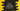# C++ Interview question : Find the sum of the series 1 + 3 + 5 + …..## Introduction

In this C++ tutorial, I will show you how to find the sum of the series 1 +3 +5…. This is a beginner level interview question. We will show you two different ways to solve it.

### Method 1 : Using a for loop :

Any problem that you need to iterate, you can use a loop. It can be a for loop, while loop or do-while loop.

For this problem, we need to find the sum of a series. The difference between two consecutive elements is 2. We will use one loop, that will run from 1 to total numbers. We will use one separate variable to store the sum. For each iteration, we will increment this sum variable or we will add the current element to this sum variable.

``````#include <iostream>
#include <iomanip>
using namespace std;
int main()
{
int total;
int sum = 1;
cout << "Enter total number : " << endl;
cin >> total;
for (int i = 2; i <= total; i++)
{
sum += 2 * i - 1;
}
cout << "Sum : " << sum << endl;
}``````

Here, the sum variable is initialized as 1. The loop runs from i = 2 to i = total. It adds 2*i - 1 on each iteration.

Suppose, we are adding the first five elements of the series :

``````i = 1, current series element is = 2*1 - 1 = 1
i = 2, current series element is = 2*2 - 1 = 3
i = 3, current series element is = 2*3 - 1 = 5
i = 4, current series element is = 2*4 - 1 = 7
``````

So, 2*i - 1 always results in the current series element. Once the loop will end, sum will hold the sum of all digits or the first total digits.

### Sample output :

``````Enter total number :
5
Sum : 25

Enter total number :
20
Sum : 400
``````

### Using the arithmetic series formula :

1 + 3 + 5…. is an arithmetic series with common difference 2. We can find out the sum of arithmetic series using the below formula :

``````(n/2)*[2a+(n−1)d]
``````

Where n is the total number of elements starting from first, a is the first element and d is a common difference. For our example, we have a = 1 and d = 2. We can take the value of n from the user as input and calculate the sum :

``````#include <iostream>
#include <iomanip>
using namespace std;
int main()
{
int n;
int sum;
cout << "Enter total number : " << endl;
cin >> n;
sum = (n/2)*(2 + (n-1)*2);
cout << "Sum : " << sum << endl;
}``````

### Output :

``````Enter total number :
10
Sum : 100

Enter total number :
15
Sum : 210
``````